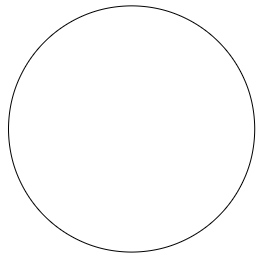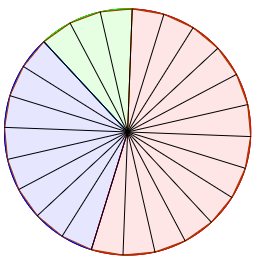### Home > ACC6 > Chapter 2 Unit 10 > Lesson CC2: 2.1.1 > Problem2-10

2-10.

Mario ordered a pizza for dinner. When it arrived, Mario quickly ate $\frac { 1 } { 8 }$ of the pizza. While Mario was getting napkins, his pet poodle ate $\frac { 1 } { 3 }$ of the pizza.

1. Draw a model of the pizza that shows the portion that has been eaten.

You should start with a circle that represents the whole pizza. A circle

Then, find what size pieces could make up one third of the pizza or one eighth of the pizza. You can do this by finding the lowest common multiple of $8$ and $3$, the denominators of the two fractions.

The LCM of $3$ and $8$ is $24$, so there should be $24$ pieces. Indicate which ones were eaten, and which ones were not. The circle is divided into 24 slices, with 13 slices red, 8 slices purple, and 3 slices green.2. Write a numerical expression to show the fraction of the pizza that is left.

Because Mario started with one whole pizza, your expression will start with one. Because Mario and his dog ate parts of the pizza, you will subtract from one the fractions of the pizza they ate.

3. About what percent of the pizza is left?

To get the percentage, you will need to have the fraction of the pizza left. You can either count the parts of the pizza left in your diagram, or simplify the expression from part (b). From there, follow the steps from the Math Notes box for Lesson 2.1.2.